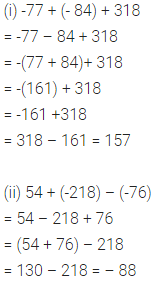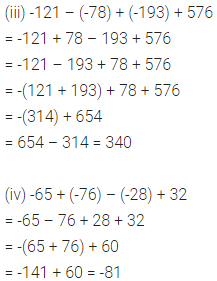# ML Aggarwal Class 6 Solutions for ICSE Maths Chapter 3 Integers Ex 3.4

## ML Aggarwal Class 6 Solutions for ICSE Maths Chapter 3 Integers Ex 3.4

Question 1.
Find the value of:
(i) 6 – 9 + 4
(ii) -5 – (-3) + 2
(iii) 7 + (-5) + (-6)
(iv) 6 – 3 – (-5)
Solution:Question 2.
Evaluate the following:
(i) -77 + (-84) + 318
(ii) 54 + (-218) – (-76)
(iii) -121 – (-78) + (-193) + 576
(iv) -65 + (-76) – (-28) + 32
Solution:Question 3.
Find the value of:
(i) 8 – 6 + (-2) – (-3) + 1
(ii) 31 + (-23) – 35 + 18 – 4 – (-3)
Solution:
(i) 8 – 6 + (-2) – (-3) + 1
= 8 – 6 – 2 + 3 + 1
=-6 – 2 + 8 + 3 + 1
= -6 – 2 + 12
=-8 + 12 = 4

(ii) 31 + (-23) – 35 + 18 – 4 – (-3)
= 31 – 23 – 35 + 18 – 4 + 3
= -23 – 35 – 4 + 31 + 18 + 3
= -23 – 35 – 4 + 52
= -62 + 52 = -10

Question 4.
Rashmi deposited ₹ 4370 in her account on Monday and then withdrew ₹ 2875 on Tuesday. Next day she deposited ₹ 1550. What was her balance on Thursday?
Solution:
Rashmi deposited in her account on Monday = ₹ 4370
Less withdrawal on Tuesday = ₹ 2875
So the Balance on Tuesday
= ₹ 4370 – ₹ 2875
= ₹ 1495
Again she deposited on Wednesday = ₹ 1550
Balance on Thursday
= ₹ 1495 + ₹ 1550 = ₹ 3045